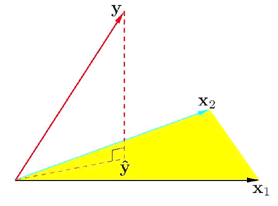# 3.2 - Point EstimatePrinter-friendly version
• The least square estimation of  $\hat{\beta}$ is:

$\hat{\beta} =(X^{T}X)^{-1}X^{T}y$

• The fitted value vector is:

$\hat{y} =X\hat{\beta}=X(X^{T}X)^{-1}X^{T}y$

• Hat matrix:

$H=X(X^{T}X)^{-1}X^{T}$

#### Geometric Interpretation

Each column of X is a vector in an N-dimensional space (not the p+1 dimensional feature vector space). Here, we take out columns in matrix X, and this is why they live in N dimensional space. Values for the same variable across all of the samples are put in a vector. I represent this input matrix as the matrix formed by the column vectors:

X = (x0, x1, ..., xp)

Here x0 is the column of 1's for the intercept term.  It turns out that the fitted output vector $\hat{y}$ is a linear combination of the column vectors xj, j = 0, 1, ... , p. Go back and look at the matrix and you will see this.

This means that $\hat{y}$ lies in the subspace spanned by xj , j = 0, 1, ... , p.

The dimension of the column vectors is N, the number of samples. Usually the number of samples is much bigger than the dimension p. The true y can be any point in this N dimensional space. What we want to find is an approximation constraint in the p+1 dimensional space such that the distance between the true y and the approximation is minimized. It turns out that the residual sum of squares is equal to the square of the Euclidean distance between y and $\hat{y}$.

$RSS(\hat{\beta})=\parallel y - \hat{y}\parallel^2$

For the optimal solution, $y-\hat{y}$ has to be perpendicular to the subspace, i.e.,  $\hat{y}$ is the projection of y on the subspace spanned by xj , j = 0, 1, ... , p.

Geometrically speaking let's look at a really simple example. Take a look at the diagram below. What we want to find is a $\hat{y}$ that lies in the hyperplane defined or spanned by x1 and x2. You would draw a perpendicular line from y to the plane to find $\hat{y}$. This comes from a basic geometric fact. In general, if you want to find some point in a subspace to represent some point in a higher dimensional space, the best you can do is to project that point to your subspace.The difference between your approximation and the true vector has to be perpendicular to the subspace.

The geometric interpretation is very helpful for understanding coefficient shrinkage and subset selection (covered in Lessons 4 and 5).